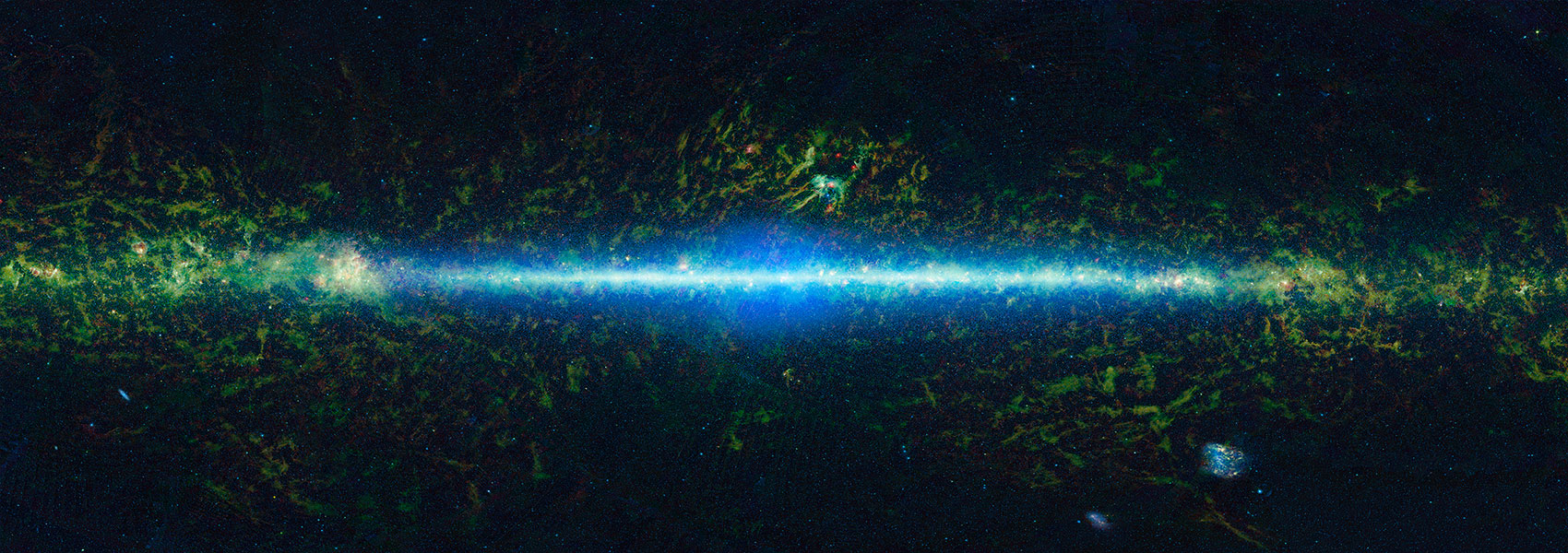# Spitzer + VLTI-GRAVITY Measure the Lens Mass of a Nearby Microlensing Event

July 2020 • 2020ApJ...897..180Z

Authors • Zang, Weicheng • Dong, Subo • Gould, Andrew • Calchi Novati, Sebastiano • Chen, Ping • Yang, Hongjing • Li, Shun-Sheng • Mao, Shude • Alton, K. B. • Brimacombe, J. • Carey, Sean • Christie, G. W. • Delplancke-Ströbele, F. • Feliz, Dax L. • Gaudi, B. Scott • Green, J. • Hu, Shaoming • Jayasinghe, T. • Koff, R. A. • Kurtenkov, A. • Mérand, A. • Minev, Milen • Mutel, Robert • Natusch, T. • Roth, Tyler • Shvartzvald, Yossi • Sun, Fengwu • Vanmunster, T. • Zhu, Wei

Abstract • We report the lens mass and distance measurements of the nearby microlensing event TCP J05074264+2447555 (Kojima-1). We measure the microlens parallax vector ${{\boldsymbol{\pi }}}_{{\rm{E}}}$ using Spitzer and ground-based light curves with constraints on the direction of lens-source relative proper motion derived from Very Large Telescope Interferometer (VLTI) GRAVITY observations. Combining this ${{\boldsymbol{\pi }}}_{{\rm{E}}}$ determination with the angular Einstein radius ${\theta }_{{\rm{E}}}$ measured by VLTI-GRAVITY observations, we find that the lens is a star with mass ${M}_{{\rm{L}}}=0.495\pm 0.063\,{M}_{\odot }$ at a distance DL = 429 ± 21 pc. We find that the blended light basically all comes from the lens. The lens-source proper motion is ${\mu }_{\mathrm{rel},\mathrm{hel}}=26.55\pm 0.36\,\mathrm{mas}\,{\mathrm{yr}}^{-1}$ , so with currently available adaptive-optics instruments, the lens and source can be resolved in 2021. This is the first microlensing event whose lens mass is unambiguously measured by interferometry + satellite-parallax observations, which opens a new window for mass measurements of isolated objects such as stellar-mass black holes.